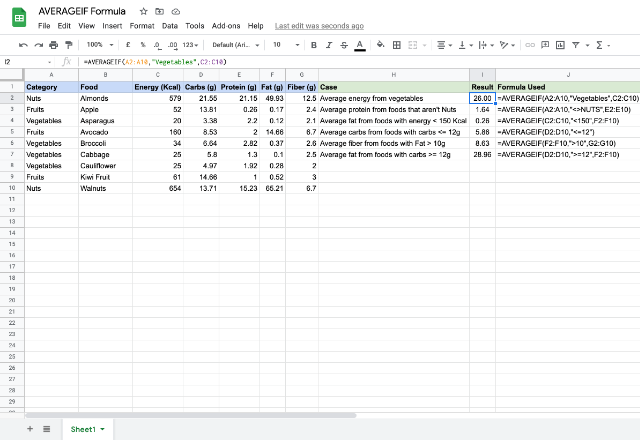# How to use the AVERAGEIF formula in Google SheetsThe AVERAGEIF formula in Google Sheets is similar to the AVERAGE formula, but with a key difference. Like the name suggests, it gives you the average of a row or column only if the value meets certain criteria. In effect, it’s the AVERAGE and IF formulas combined into one handy formula. So it’s a pretty useful tool that will save you time and get your spreadsheet working more efficiently.

### Syntax

AVERAGEIF(criteria_range, criterion, [average_range])

• criteria_range – this is the address reference to a range of cells against which the formula checks for the criterion.
• criterion – is the condition or test that is used to qualify the criteria_range cells for averaging. There are six types of comparisons in general, as listed below. While we can compare both numbers and text using the first two operators, we can only compare numbers using the last four operators.
• Equals (=)
• Not equal to (<>)
• Greater than (>)
• Greater than or equal to (>=)
• Less than (<)
• Less than or equal to (<=)
• average_range – is an optional address reference to the range of cells that the AVERAGEIF formula considers for averaging. If we do not specify this parameter, the formula averages criteria_range.

### How to use the AVERAGEIF formula

Below is a sample of nutritional information from a select set of foods. Let’s try a few examples to understand how the formula works in practise.We have sample data populating cells A1 through to G10. Notice that the first two cases are text comparisons while the last four cases are number based comparisons.

Let’s look at the first case. The first parameter is the criteria_range, which is A2:A10. The criterion is “Vegetables”. And the average_range is C2:C10. So, the AVERAGEIF formula checks for instances of “Vegetables” within the range A2:A10, then averages the values from the range C2:C10 that are in the rows where it finds “Vegetables”. Now, let’s look at the case in row 5. You’ll see that we did not specify the last parameter. In this case the formula is doing both criteria checking and value averaging on the criteria_range itself i.e. D2:D10.

While the AVERAGEIF returns the average of values satisfying a single criterion, the AVERAGEIFS formula gives us the average of values subject to multiple criteria. Learn how to use the AVERAGEIFS formula in Google Sheets.